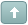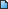Error

# HitTest a polyline

#1 Posted : Friday, September 14, 2007 12:16:31 PM(UTC)
Hi,
how can I get the segment of a polyline when I hittested it?
I'm using LT15 with VB.NET
Thanks!

 Try the latest version of LEADTOOLS for free for 60 days by downloading the evaluation: https://www.leadtools.com/downloadsWanna join the discussion? Login to your LEADTOOLS Support accountor Register a new forum account.#2 Posted : Sunday, September 16, 2007 1:08:07 AM(UTC)
Hello,

The following code shows how to get the segment of a AnnPolylineObject when you Hittest it:
+------------------+

Private Sub RasterImageViewer1_MouseMove(ByVal sender As Object, ByVal e As System.Windows.Forms.MouseEventArgs) Handles RasterImageViewer1.MouseMove
' perform hit-testing and update the status bar
If Not RasterImageViewer1.Image Is Nothing Then
If Not AutomationAnn.Container Is Nothing Then

Dim pt As AnnPoint = New AnnPoint(e.X, e.Y, AnnUnit.Pixel)
Dim obj As AnnObject = AutomationAnn.Container.HitTest(pt, 2)
Dim PolylineObject As AnnPolylineObject
Dim i As Integer

If Not obj Is Nothing Then

Text = String.Format("Type: {0}, Name: {1}, Value: {2}", obj.GetType().Name, obj.Name, obj.HitTest(pt, 2))

If TypeOf (obj) Is AnnPolylineObject Then
PolylineObject = obj
Text = pt.X.ToString() _
+ " , " + pt.Y.ToString()

For i = 0 To PolylineObject.Points.Count - 1
Dim P1 As New AnnPoint
P1 = PolylineObject.Points(i)

Dim P2 As New AnnPoint
If i = PolylineObject.Points.Count - 1 Then
P2 = P1
Else
P2 = PolylineObject.Points(i + 1)
End If

'Now, check if the pt point between p1 and p2
'If pt is between p1 and p2, then you can
'get the segment by using the
'points p1 and p2
Next

End If
Else
Text = String.Empty
End If
End If
End If

End Sub
+------------------+

Please let me know if this helps.

Thanks,

#3 Posted : Monday, September 17, 2007 4:28:54 AM(UTC)
Hi,
but my question is how can do for determinig if pt is between p1 and p2, I just know the use of PolylineObject collection.
Thanks!!!

#4 Posted : Tuesday, September 18, 2007 2:52:30 AM(UTC)
Hello,

The idea is to loop through the PolylineObject Points (like my code does), and compare the hit point with every segment (every 2 points).

You should be able to determine if a hit point is in the segment using basic geometry calculations (comparing end points and slopes).
In particular, if the X,Y values of the hit point are between the X,Y values of the 2 end points, and the slope (slope = (Point2.Y – Point1.Y) / (Point2.X – Point1.X)) of the segment is equal to the slope of line connecting one end point to the hit point, this means they're all on the same line.

Thanks,

#5 Posted : Sunday, September 4, 2011 11:10:01 PM(UTC)

Hi,

I did the same thing, but I am not getting the expected result.

I have two approaches for the given problem.

1. Find the slope of two lines and match, they should be same if pointx lies on a line (StartPoint,EndPoint)

2. Find the slope and intercept. Then satify the straightline equation Y= mX +c

Please find my code, and suggest If I am doing anything wrong

private void DoHitTest(MouseEventArgs e)
{
float tolerance = 0.001f;
AnnPoint pointTobeSearched = new AnnPoint(
(e.X + Viewer.ScrollPosition.X) / (float)Viewer.CurrentYScaleFactor,
(e.Y + Viewer.ScrollPosition.Y) / (float)Viewer.CurrentYScaleFactor);

for (int i = 0; i < (PickedPoints.Count - 2); i++)
{
AnnPoint Point1 = this.PickedPoints[i];
AnnPoint Point2 = this.PickedPoints[i + 1];

float slopeFullLine = (Point2.Y - Point1.Y) / (Point2.X - Point1.X);
float slopeTillHitPoint = (pointTobeSearched.Y - Point1.Y) / (pointTobeSearched.X - Point1.X);

//below not working (Comparison of both slopes.
if ((slopeFullLine - slopeTillHitPoint) <= tolerance)
{
IndexToBeInserted = i;
break;
}

if ((Point2.X - Point1.X) <= tolerance)  // Vertical line.
{
this.IndexToBeInserted = i;
}

float M = (Point2.Y - Point1.Y) / (Point2.X - Point1.X); // Slope
float C = -(M * Point1.X) + Point1.Y; // Y intercept

// Checking if (x, y) is on the line passing through the end points. (not working)
if (Math.Abs(pointToBeSearched.Y - ((M * pointToBeSearched.X) + C)) <= tolerance)
{
this.IndexToBeInserted = i;
}

}

#6 Posted : Monday, September 5, 2011 5:53:18 AM(UTC)
If you're having difficulty with the calculations, I can try to help you if you put your code in a small test project (not your full application) and send it to me. You can either post the test project here or email it to support@leadtools.com
If you post it here, do not use the preview feature when attaching.

In either case, make sure to put all attachments in a ZIP or RAR file to ensure our server delivers them correctly.

#7 Posted : Monday, September 5, 2011 9:23:53 AM(UTC)

I am using a different approach now. I am trying to use IsOutlineVisible Method of Graphics path. But still I am failed :(

I am creating a path using two adjacent points. Then finding the hit point in the given path. Please find my code below and please help me out..

if (e.Button == MouseButtons.Left)

{

CurvePolyLine obj = ObjectTemplate as CurvePolyLine;

if (obj.CurrentMode == Common.FrmCurveEditorMode.EditCurve)

{

PointF hitPoint = new PointF(e.X,e.Y);

for (int i = 0; i < (obj.PickedPoints.Count - 2); i++)

{

GraphicsPath graphicsPath = new GraphicsPath();

AnnPoint annPoint1 = obj.PickedPoints[i];

AnnPoint startPoint = annPoint1.ConvertTo(Container.UnitConverter, AnnUnit.Pixel);

AnnPoint annPoint3 = obj.PickedPoints[i+1];

AnnPoint endPoint = annPoint3.ConvertTo(Container.UnitConverter, AnnUnit.Pixel);

//Needs to be created

Matrix m = new Matrix();

float width = obj.Pen.Width.Converted(Container.UnitConverter,AnnUnit.Pixel);

Pen p = new Pen(System.Drawing.Color.Black,width);

//graphicsPath.Transform(m);

bool b = graphicsPath.IsOutlineVisible(hitPoint, p);

graphicsPath.Widen(p);

p.Dispose();

if (b)

{

MessageBox.Show("Found");

}

if (graphicsPath.IsVisible(hitPoint))

{

MessageBox.Show("Found");

}

}

}

#8 Posted : Monday, September 5, 2011 10:54:04 AM(UTC)

Hi

I am attaching a sample project with my both approaches

Approach 1: Satisfying the line equation.

Approach 2: Using IsOutlineVisible of Graphics Path

Thanks

Raj

File Attachment(s):SampleTestApplication.zip (48kb) downloaded 30 time(s).

#9 Posted : Tuesday, September 6, 2011 5:18:26 AM(UTC)
Raj,

I did it using the slope approach. I covered most cases except the vertical line. I am attaching the modified project.

Thanks,SampleTestApplication.zip (48kb) downloaded 30 time(s).